Courses

# Test: Kinetic Theory - From Past 28 Years Questions

## 19 Questions MCQ Test Physics 28 Years Past year papers for NEET/AIPMT Class 11 | Test: Kinetic Theory - From Past 28 Years Questions

Description
This mock test of Test: Kinetic Theory - From Past 28 Years Questions for NEET helps you for every NEET entrance exam. This contains 19 Multiple Choice Questions for NEET Test: Kinetic Theory - From Past 28 Years Questions (mcq) to study with solutions a complete question bank. The solved questions answers in this Test: Kinetic Theory - From Past 28 Years Questions quiz give you a good mix of easy questions and tough questions. NEET students definitely take this Test: Kinetic Theory - From Past 28 Years Questions exercise for a better result in the exam. You can find other Test: Kinetic Theory - From Past 28 Years Questions extra questions, long questions & short questions for NEET on EduRev as well by searching above.
QUESTION: 1

### Two containers A and B are partly filled with water and closed. The volume of A is twice that of B and it contains half the amount of water in B. If both are at the same temperature, the water vapour in the containers will have pressure in the ratio of 

Solution:

Vapour pressure  does not depend on the amount of substance. It depends on the temperature alone.

QUESTION: 2

### At constant volume, temperature is increased then

Solution:

As the temperature increases, the average velocity increases. So, the collisions are faster.

QUESTION: 3

### A polyatomic gas with n degrees of freedom has a mean energy per molecule given by 

Solution:

According to law of equipartition of energy, the energy per degree of freedom is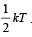For a polyatomic gas with n degrees of freedom, the mean energy per molecule  =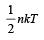QUESTION: 4

For a certain gas the ratio of specific heats is given to be γ = 1.5. For this gas 

Solution: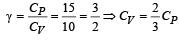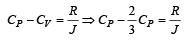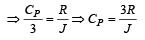QUESTION: 5

According to kinetic theory of gases, at absolute zero temperature 

Solution:

The average kinetic energy of a gas molecular is directly eruptional to absolute temperature only; this implies that all molecular motion stops if the temperature is reduced to absolute zero.

QUESTION: 6

One mole of an ideal monoatomic gas requires 207 J heat to raise the temperature by 10 K when heated at constant pressure. If the same gas is heated at constant volume to raise the temperature by the same 10 K, the heat required is [Given the gas constant R = 8.3 J/ mol. K] 

Solution: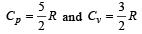We know that  Qv = nCvΔT and Qp = nCpΔT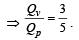Given Qp = 207 J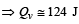QUESTION: 7

Relation between pressure (P) and energy (E) of a gas is 

Solution: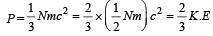QUESTION: 8

Three containers of the same volume contain three different gases. The masses of the molecules are m1, m2 and m3 and the number of molecules in their respective containers are N1, N2 and N3. The gas pressure in the containers are P1, P2 and P3 respectively. All the gases are now mixed and put in one of these containers.The pressure P of the mixture will be 

Solution:

According to Dalton’s law of partial pressures, we have  P = P1 + P2 + P3

QUESTION: 9

The number of translational degrees of freedom for a diatomic gas is 

Solution:

Number of translational degrees of freedom are same for all types of gases.

QUESTION: 10

The pressure of a gas is raised from 27°C to 927°C.The root mean square speed is 

Solution: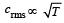As temperature increases from 300 K to 1200 K that is four times, so, crms will be doubled.

QUESTION: 11

The equation of state, corresponding to 8g of O2 is

Solution:

8g of oxygen is equivalent to (1/4) mole.

QUESTION: 12

If Cs be the velocity of sound in air and C be the r.m.s velocity, then 

Solution:

Velocity of sound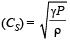R.M.S. velocity of gas molecules =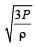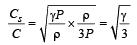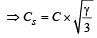QUESTION: 13

At 0 K, which of the following properties of a gas will be zero? 

Solution:

At 0 K, molecular motion stops. Hence, kinetic energy of molecules becomes zero.

QUESTION: 14

The degree of freedom of a molecule of a triatomic ga s is

Solution:

No. of degree of freedom = 3 K – N where K is no. of atom and N is the number of relations between atoms. For triatomic gas, K = 3, N = 3C2 = 3
No. of degree of freedom = 3 (3) – 3 = 6

QUESTION: 15

The equation of state for 5 g of oxygen at a pressure P and temperature T, when occupying a volume V, will be 

Solution: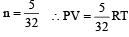[∵ PV = nRT]

QUESTION: 16

At 10° C the value of the density of a fixed mass of an ideal gas divided by its pressure is x. At 110°C this ratio is: 

Solution:

Let the mass of the gas be m.
At a fixed temperature and pressure, volume is fixed.
Density of the gas,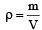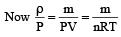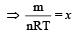(By question)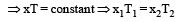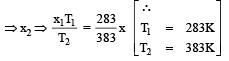QUESTION: 17

In the given (V – T) diagram, what is the relation between pressure P1 and P2 ? [NEET 2013]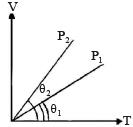Solution:

P1 > P2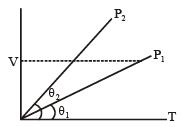As V = constant ⇒ P ∝ T
Hence from V–T graph P1 > P2

QUESTION: 18

The amount of heat energy required to raise the temperature of 1g of Helium at NTP, from T1K to T2K is [NEET 2013]

Solution:

From first law of thermodynamics ΔQ = ΔU + ΔW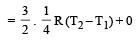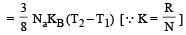QUESTION: 19

In a vessel, the gas is at a pressure P. If the mass of all the molecules is halved and their speed is doubled, then the resultant pressure will be [NEET Kar. 2013]

Solution: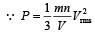When mass is halved and speed is doubled then Resultant pressure,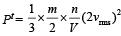= 2 P.